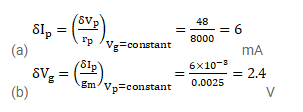# The plate resistance of a triode is 8k Ω and the transconductance is 2.5millimho.

Question:

The plate resistance of a triode is $8 \mathrm{k}^{\Omega}$ and the transconductance is $2.5$ millimho. (a) If the plate voltage is increased by $48 \mathrm{~V}$, and the grid voltage is kept constant, what will be the increase in the plate current? (b) With plate voltage kept constant at this increased value, how much should the grid voltage be decreased in order to bring the plate current back to its initial value?

Solution: